Chapter 12, Problem 12PS

Chapter
Section
Textbook Problem

A unit cell of cesium chloride is illustrated in Figure 12.8. The density of the solid is 3.99 g/cm3, and the radius of the Cl− ion is 181 pm. Calculate the radius of the Cs+ ion in the center of the cell. (Assume that the Cs+ ion touches all of the comer Cl− ions.)

Interpretation Introduction

Interpretation:

The radius of the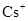ion has to be calculated.

Concept introduction:

The volume of the atom is given below,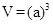The density of the unit cell is calculated as follows,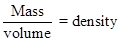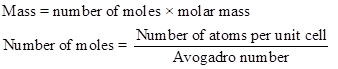To calculate the radius of the ion is given below,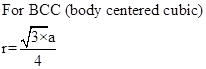Explanation

Therefore,

Still sussing out bartleby?

Check out a sample textbook solution.

See a sample solution

The Solution to Your Study Problems

Bartleby provides explanations to thousands of textbook problems written by our experts, many with advanced degrees!

Get Started

Partially completed proteins are not held for completion at a later time when the diet may improve. T F

Nutrition: Concepts and Controversies - Standalone book (MindTap Course List)

How do eating disorders affect health?

Understanding Nutrition (MindTap Course List)

Distinguish between acids and bases. What are their properties?

Biology: The Dynamic Science (MindTap Course List)

What are genomes?

Human Heredity: Principles and Issues (MindTap Course List)